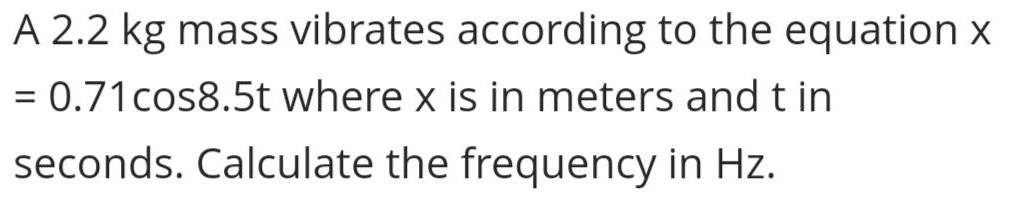Question:

# A 2.2 kg mass vibrates according to the equation x = 0.71

Last updated: 8/7/2022A 2.2 kg mass vibrates according to the equation x = 0.71 cos8.5t where x is in meters and t in seconds. Calculate the frequency in Hz.